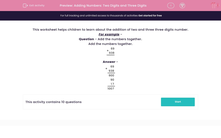# Adding Numbers: Two Digits and Three Digits

In this worksheets, students add together a two-digit number and a three digit number using a formal column method.This content is premium and exclusive to EdPlace subscribers.Key stage:  KS 2

Curriculum topic:   Maths and Numerical Reasoning

Curriculum subtopic:   Mixed Problems

Difficulty level:#### Worksheet Overview

This worksheet helps children to learn about the addition of two and three three digits number.

For example -

Question - Add the numbers together.

 + 6 9 9 3 8